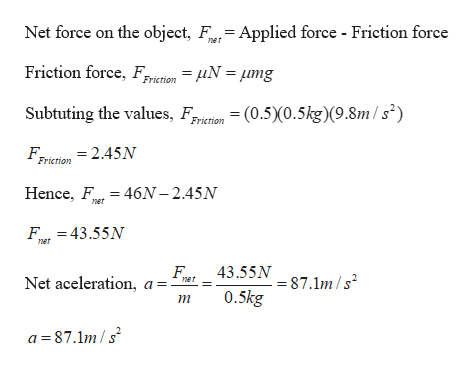A force of 46N is applied horizontally to a box with a mass of 0.5kg. The block sits on a horizontal table where the coefficient of friction between the box and the table is .5.What is the acceleration of the box? (In m/s^2)

Question

A force of 46N is applied horizontally to a box with a mass of 0.5kg. The block sits on a horizontal table where the coefficient of friction between the box and the table is .5.

What is the acceleration of the box? (In m/s^2)

Step 1

Given:

Mass of the object = 0.5 kg

Coefficient of friction = 0.5

Value of applied force = 46 N

Step 2

Calculating the accelerat...help_outlineImage TranscriptioncloseNet force on the object, F= Applied force - Friction force net Friction force, Friction = fiN = mg Subtuting the values, iion (0.5)0.5kg)(9.8m/s2) Friction F, Friction = 2.45N Hence, F 46N-2.45N net F43.55N net 43.55N 7.1m/s Net aceleration ner 0.5kg т a 87.1m/ fullscreen

Want to see the full answer?

See Solution

Want to see this answer and more?

Our solutions are written by experts, many with advanced degrees, and available 24/7

See Solution
Tagged in

Newtons Laws of Motion